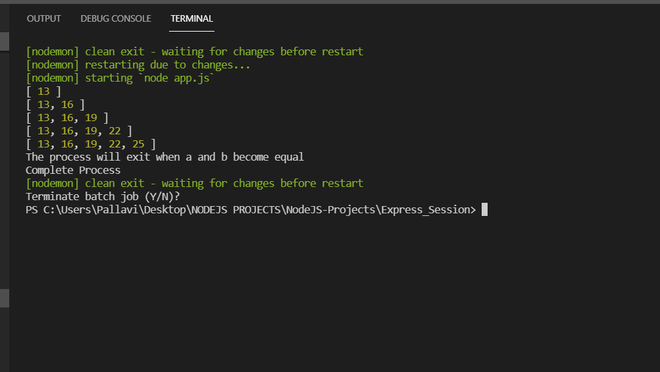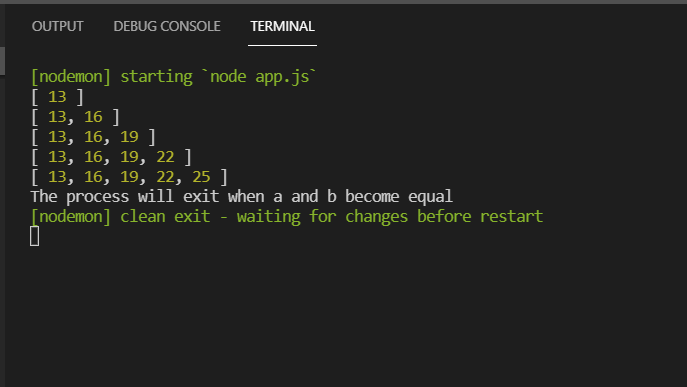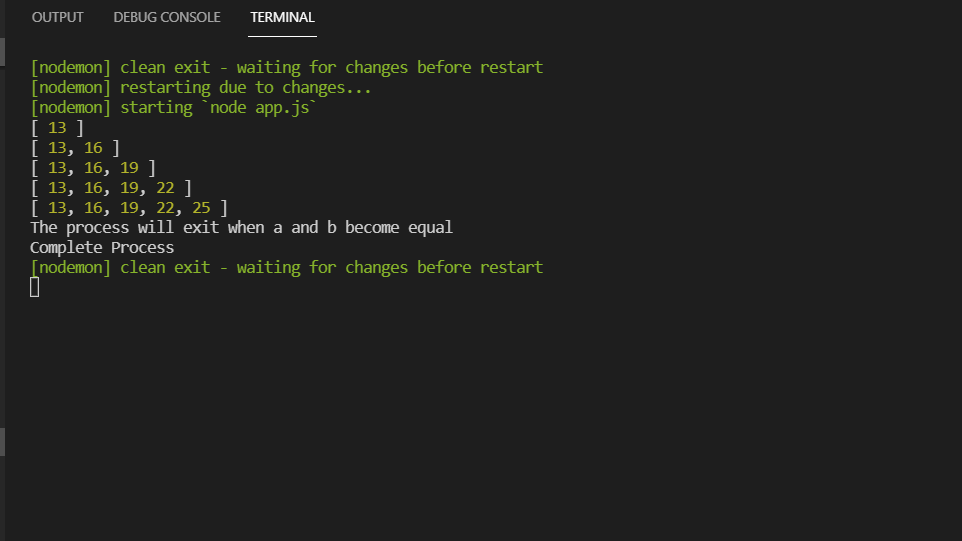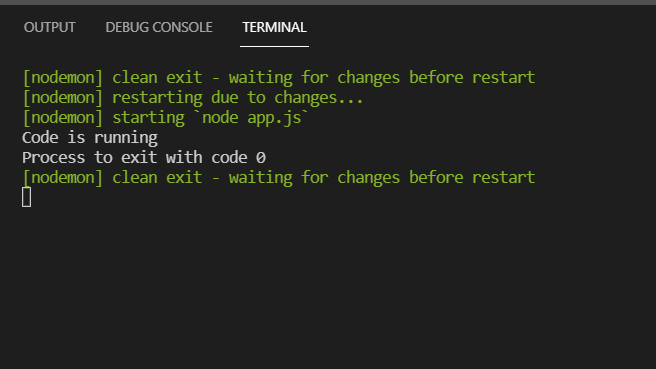Related Articles

# How to exit process in Node.js ?

• Difficulty Level : Medium
• Last Updated : 25 Jun, 2021

In this article, we will see how to exit in NodeJS application. There are different types of methods to exit in Nodejs application, here we have discussed the following four methods.

Method 1: Using ctrl+C key: When running a program of NodeJS in the console, you can close it with ctrl+C directly from the console with changing the code shown below:Method 2: Using process.exit() Function: This function tells Node.js to end the process which is running at the same time with an exit code. By calling this function Node.js will force the current process that’s running to exit as soon as possible.

Syntax:

`process.exit(code)`

Parameter: This function accepts single parameter as mentioned above and described below:

• code: It can be either 0 or 1 value. Here 0 means end the process without any kind of failure and 1 means end the process with some failure.

## app.js

 `// An empty array.``var` `arr = [];`` ` `// Variable a and b``var` `a = 8;``var` `b = 2;`` ` `// While condition to run loop``// infinite times``while` `(a != 0 || b != 0) {`` ` `    ``// Increment then value``    ``// of a and b  ``    ``a = a + 1;``    ``b = b + 2;`` ` `    ``// Push the sum of a and b``    ``// into array``    ``arr.push(a + b);`` ` `    ``// If a and b become equal it``    ``// will exit the process``    ``if` `(a == b) {``        ``console.log(``"The process will "``        ``+ ``"exit when a and b become equal"``);``        ``process.exit(0);`` ` `        ``console.log(``"Complete Process"``)``    ``}`` ` `    ``//  It will print the result when``    ``// a and is not equal``    ``else` `{``        ``console.log(arr);``    ``}``}`

Output:Method 3: Using process.exitCode variable: Another method is used to set the process.exitCode value which will allows the Node.js program to exit on its own without leaving further calls for the future. This method is safer and will result in less issues in your Node.js code.

## app.js

 `// An empty array.``var` `arr = [];`` ` `// Variable a and b``var` `a = 8;``var` `b = 2;`` ` `// While condition to run``// loop infinite times``while` `(a > b) {`` ` `    ``// Increment then value``    ``// of a and b``    ``a = a + 1;``    ``b = b + 2;`` ` `    ``// Push the sum of a and``    ``// b into array``    ``arr.push(a + b);`` ` `    ``// If a and b become equal``    ``// it will exit the process``    ``if` `(a == b) {``        ``console.log(``"The process will "``        ``+ ``"exit when a and b become equal"``);``        ``process.exitCode = 0;``        ``console.log(``"Complete Process"``)``    ``}`` ` `    ``//  It will print the result when``    ``// a and is not equal``    ``else` `{``        ``console.log(arr);``    ``}``}`

Output:Method 4: Using process.on() Function: The Process object is a global variable that let us manage the current Node.js, process will exit when it reaches the end of the line of the code we do have to use require for the process because it is automatically present in the NodeJS.

## app.js

 `console.log(``'Code is running'``);`` ` `process.on(``'exit'``, ``function` `(code) {``    ``return` `console.log(`Process to exit ``with` `code \${code}`);``});`

Output:My Personal Notes arrow_drop_up